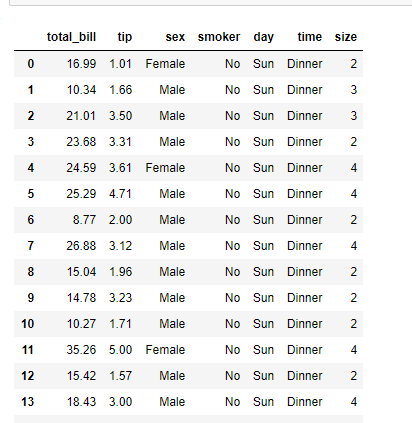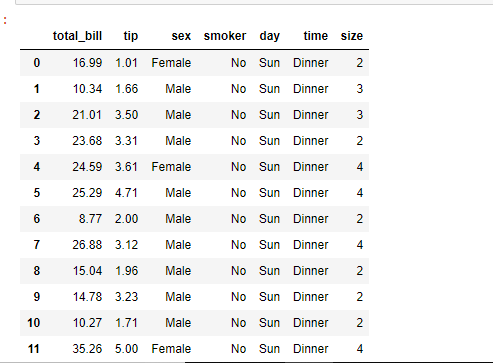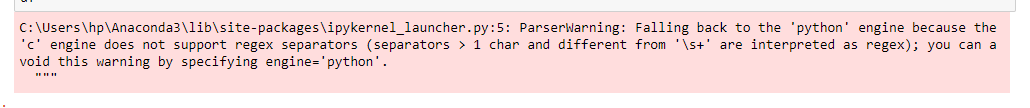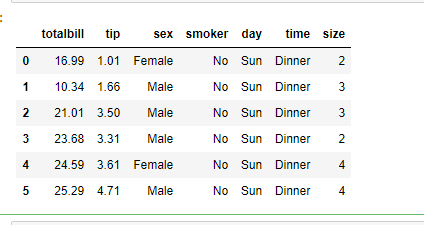GeeksforGeeks App
Open AppBrowser
Continue

# How to read a CSV file to a Dataframe with custom delimiter in Pandas?

Python is a good language for doing data analysis because of the amazing ecosystem of data-centric python packages. pandas package is one of them and makes importing and analyzing data so much easier.
Here, we will discuss how to load a csv file into a Dataframe. It is done using a pandas.read_csv() method. We have to import pandas library to use this method.

Syntax: pd.read_csv(filepath_or_buffer, sep=’, ‘, delimiter=None, header=’infer’, names=None, index_col=None, usecols=None, squeeze=False, prefix=None, mangle_dupe_cols=True, dtype=None, engine=None, converters=None, true_values=None, false_values=None, skipinitialspace=False, skiprows=None, nrows=None, na_values=None, keep_default_na=True, na_filter=True, verbose=False, skip_blank_lines=True, parse_dates=False, infer_datetime_format=False, keep_date_col=False, date_parser=None, dayfirst=False, iterator=False, chunksize=None, compression=’infer’, thousands=None, decimal=b’.’, lineterminator=None, quotechar='”‘, quoting=0, escapechar=None, comment=None, encoding=None, dialect=None, tupleize_cols=None, error_bad_lines=True, warn_bad_lines=True, skipfooter=0, doublequote=True, delim_whitespace=False, low_memory=True, memory_map=False, float_precision=None)

Some Useful parameters are given below :

This method uses comma ‘, ‘ as a default delimiter but we can also use a custom delimiter or a regular expression as a separator.
Example 1 : Using the read_csv() method with default separator i.e. comma(, )

## Python3

 `# Importing pandas library``import` `pandas as pd` `# Using the function to load``# the data of example.csv``# into a Dataframe df``df ``=` `pd.read_csv(``'example1.csv'``)` `# Print the Dataframe``df`

Output:Example 2: Using the read_csv() method with ‘_’ as a custom delimiter.

## Python3

 `# Importing pandas library``import` `pandas as pd` `# Load the data of example.csv``# with '_' as custom delimiter``# into a Dataframe df``df ``=` `pd.read_csv(``'example2.csv'``,``                   ``sep ``=` `'_'``,``                   ``engine ``=` `'python'``)` `# Print the Dataframe``df`

Output:Note:While giving a custom specifier we must specify engine=’python’ otherwise we may get a warning like the one given below:Example 3 : Using the read_csv() method with tab as a custom delimiter.

## Python3

 `# Importing pandas library``import` `pandas as pd` `# Load the data of example.csv``# with tab as custom delimiter``# into a Dataframe df``df ``=` `pd.read_csv(``'example3.csv'``,``                   ``sep ``=` `'\t'``,``                   ``engine ``=` `'python'``)` `# Print the Dataframe``df`

Output:Example 4 : Using the read_csv() method with regular expression as custom delimiter.
Let’s suppose we have a csv file with multiple type of delimiters such as given below.

totalbill_tip, sex:smoker, day_time, size
16.99, 1.01:Female|No, Sun, Dinner, 2
10.34, 1.66, Male, No|Sun:Dinner, 3
21.01:3.5_Male, No:Sun, Dinner, 3
23.68, 3.31, Male|No, Sun_Dinner, 2
24.59:3.61, Female_No, Sun, Dinner, 4
25.29, 4.71|Male, No:Sun, Dinner, 4

To load such file into a dataframe we use regular expression as a separator.

## Python3

 `# Importing pandas library``import` `pandas as pd` `# Load the data of example.csv``# with regular expression as``# custom delimiter into a``# Dataframe df``df ``=` `pd.read_csv(``'example4.csv'``,``                   ``sep ``=` `'[:, |_]'``,``                   ``engine ``=` `'python'``)` `# Print the Dataframe``df`

Output:My Personal Notes arrow_drop_up